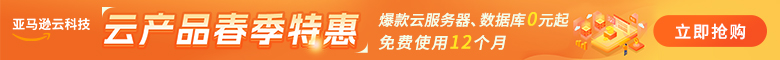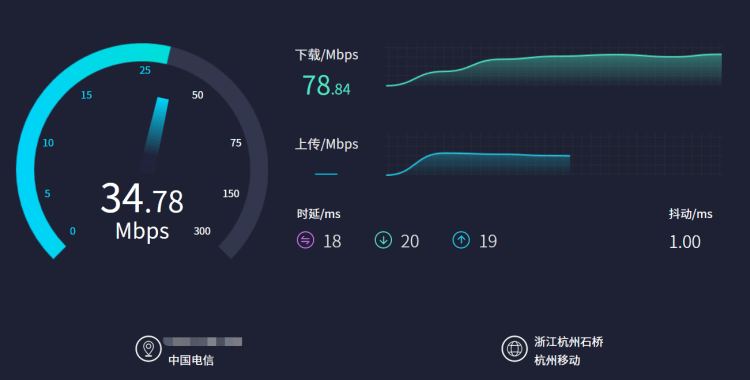# 500兆光纤网速是多少？网络软件测速速度多少10M网速：

10Mbps =10*1024 = 10240 Kb/s

=10*1024*1024 = 10485760 b/s

=10*1024*1024/8 = 1310720 B/s

=10*1024/8 = 1280 KB/s

=10/8 = 1.25 MB/s

100M网速：

100Mbps =100*1024 = 102400 Kb/s

=100*1024*1024 = 104857600 b/s

=100*1024*1024/8 = 13107200 B/s

=100*1024/8 = 12800 KB/s

=100/8 = 12.5 MB/s

200M网速：

200Mbps =200*1024 = 204800 Kb/s

=200*1024*1024 = 209715200 b/s

=200*1024*1024/8 = 26214400 B/s

=200*1024/8 = 25600 KB/s

=200/8 = 25 MB/s